Test: Surface Area & Volumes- Assertion & Reason Type Questions

# Test: Surface Area & Volumes- Assertion & Reason Type Questions - EmSAT Achieve

Test Description

## 10 Questions MCQ Test Mathematics for EmSAT Achieve - Test: Surface Area & Volumes- Assertion & Reason Type Questions

Test: Surface Area & Volumes- Assertion & Reason Type Questions for EmSAT Achieve 2023 is part of Mathematics for EmSAT Achieve preparation. The Test: Surface Area & Volumes- Assertion & Reason Type Questions questions and answers have been prepared according to the EmSAT Achieve exam syllabus.The Test: Surface Area & Volumes- Assertion & Reason Type Questions MCQs are made for EmSAT Achieve 2023 Exam. Find important definitions, questions, notes, meanings, examples, exercises, MCQs and online tests for Test: Surface Area & Volumes- Assertion & Reason Type Questions below.
Solutions of Test: Surface Area & Volumes- Assertion & Reason Type Questions questions in English are available as part of our Mathematics for EmSAT Achieve for EmSAT Achieve & Test: Surface Area & Volumes- Assertion & Reason Type Questions solutions in Hindi for Mathematics for EmSAT Achieve course. Download more important topics, notes, lectures and mock test series for EmSAT Achieve Exam by signing up for free. Attempt Test: Surface Area & Volumes- Assertion & Reason Type Questions | 10 questions in 20 minutes | Mock test for EmSAT Achieve preparation | Free important questions MCQ to study Mathematics for EmSAT Achieve for EmSAT Achieve Exam | Download free PDF with solutions
 1 Crore+ students have signed up on EduRev. Have you?
Test: Surface Area & Volumes- Assertion & Reason Type Questions - Question 1

### Direction: In the following questions, a statement of Assertion is given followed by a corresponding statement of Reason just below it. Of the statements, mark the correct answer asAssertion : If diameter of a sphere is decreased by 25%, then its curved surface area is decreased by 43.75%.Reason : Curved surface area is increased when diameter decreases

Detailed Solution for Test: Surface Area & Volumes- Assertion & Reason Type Questions - Question 1
Let the diameter of sphere be 2r.

Decreased diameter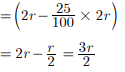New diameter = 3r/2

Curved surface area of sphere = 4πr2

New curved surface area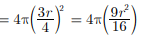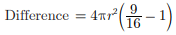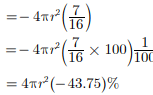Curved surface area is decreased by 43.75%.

As if diameter is decreased, curved surface area is decreased.

Test: Surface Area & Volumes- Assertion & Reason Type Questions - Question 2

### Direction: In the following questions, a statement of Assertion is given followed by a corresponding statement of Reason just below it. Of the statements, mark the correct answer asAssertion : The external dimensions of a wooden box are 18 cm, 10 cm and 6 cm respectively and thickness of the wood is 15 mm, then the internal volume is 765 cm3.Reason : If external dimensions of a rectangular box be l, b and h and the thickness of its sides be x , then its internal volume is (l - 2x) (b - 2x) (h - 2x).

Detailed Solution for Test: Surface Area & Volumes- Assertion & Reason Type Questions - Question 2
Length of box = 18 cm

Width of box = 10 cm

Height of box = 6 cm

thickness of box = 5 mm = 1/2 cm

Internal length, width, height of the box is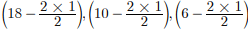Internal volume of box = 17 x 9 x 5

= 765 cm3

Test: Surface Area & Volumes- Assertion & Reason Type Questions - Question 3

### Direction: In the following questions, a statement of Assertion is given followed by a corresponding statement of Reason just below it. Of the statements, mark the correct answer asAssertion : The total surface area of a cone whose radius is r/2 and slant height 2l is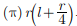Reason : Total surface area of cone is (π)r(l+r) where r is radius and l is the slant height of the cone.

Detailed Solution for Test: Surface Area & Volumes- Assertion & Reason Type Questions - Question 3
We have to calculate the total surface area of the cone whose radius is r/2 and slant height is 2l.

We know that the total surface area of a cone with radius ‘r’ and slant height ‘l’ is πr(r + l).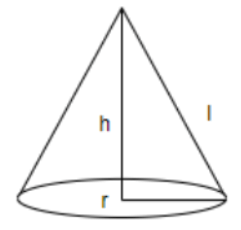We know that the radius of the given cone is r/2 and its slant height is 2l.

Substituting these values in the formula of calculating the total surface area of the cone, the total surface area of the given cone is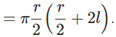Simplifying the above expression, the total surface area of the cone is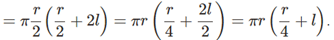Hence, the total surface area of the cone whose radius is r/2 and slant height is 2l is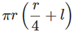Test: Surface Area & Volumes- Assertion & Reason Type Questions - Question 4

Direction: In the following questions, a statement of Assertion is given followed by a corresponding statement of Reason just below it. Of the statements, mark the correct answer as

Assertion : A cone is a solid figure.

Reason : A cone is generated when rectangular sheet is rotated about its axis

Detailed Solution for Test: Surface Area & Volumes- Assertion & Reason Type Questions - Question 4
Assertion is true.

A cone is generated when a right angled triangle is rotated about its base. So, the reason is false.

Test: Surface Area & Volumes- Assertion & Reason Type Questions - Question 5

Direction: In the following questions, a statement of Assertion is given followed by a corresponding statement of Reason just below it. Of the statements, mark the correct answer as

Assertion : In a cylinder, if radius is halved and height is doubled, the volume will be halved.

Reason : In a cylinder, radius is doubled and height is halved, curved surface area will be same.

Detailed Solution for Test: Surface Area & Volumes- Assertion & Reason Type Questions - Question 5
Let r and h be radius and height of the cyclinder,

then C.S.A. = 2πrh Now, radius is doubled and height is halved.

∴ New radius = 2r and new height = h/2

New C.S.A. = 2π × 2r × h/2 = 2πrh .

Test: Surface Area & Volumes- Assertion & Reason Type Questions - Question 6

Direction: In the following questions, a statement of Assertion is given followed by a corresponding statement of Reason just below it. Of the statements, mark the correct answer as

Assertion : If the inner dimensions of a cuboidal box are 50 cm x 40 cm x 30 cm , then the length of the longest rod that can be placed in the box is 50√2 cm.

Reason : The line joining opposite corners of a cuboid is called its diagonal.

Also, length of longest rod = length of diagonal.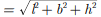Detailed Solution for Test: Surface Area & Volumes- Assertion & Reason Type Questions - Question 6
Length of the diagonal is the length of the longest rod that can be placed in the cuboid.
Test: Surface Area & Volumes- Assertion & Reason Type Questions - Question 7

Direction: In the following questions, a statement of Assertion is given followed by a corresponding statement of Reason just below it. Of the statements, mark the correct answer as

Assertion : A shot put is a metallic sphere of radius 4 cm. If the density of the metal is 10 g per cm3. then the mass of the shot put is 2 kg.

Reason : Volume of sphere of radius r is 4/3 πr3.

Detailed Solution for Test: Surface Area & Volumes- Assertion & Reason Type Questions - Question 7
Volume of metallic sphere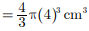Density of metal 10g cm3

Mass of shot put = Density x Volume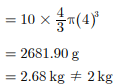Test: Surface Area & Volumes- Assertion & Reason Type Questions - Question 8

Direction: In the following questions, a statement of Assertion is given followed by a corresponding statement of Reason just below it. Of the statements, mark the correct answer as

Assertion : An edge of a cube measures r cm. If the largest possible right circular cone is cut out of this cube, then the volume of the cone is 1/3 πr3.

Reason : Volume of the cone is given by 1/3 πr2h, where r is the radius of the base and h is the height of the cone.

Detailed Solution for Test: Surface Area & Volumes- Assertion & Reason Type Questions - Question 8
Height of the cone = r cm.

Diameter of the base = r cm.

Therefore, volume of the cone = 1/3 π(r/2)2.r

= 1/12 πr3

Test: Surface Area & Volumes- Assertion & Reason Type Questions - Question 9

Direction: In the following questions, a statement of Assertion is given followed by a corresponding statement of Reason just below it. Of the statements, mark the correct answer as

Assertion : Into a circular drum of radius 4.2 m and height 3.5 m, the number of full bags of wheat can be emptied, if the space required for wheat in each bag is 2.1 cu. m, is 92 bags nearly.

Reason : Volume of circular drum is 1/3 πr2h where r is radius and h is height of drum.

Detailed Solution for Test: Surface Area & Volumes- Assertion & Reason Type Questions - Question 9
Radius of circular drum, r = 4 2. m

Height of circular drum, h = 3 5. m

Volume of circular drum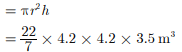Space occupied by 1 wheat bag = 2 1. m3

Number of wheat bags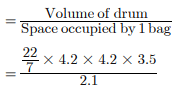= 92 4. = 92 nearly

Test: Surface Area & Volumes- Assertion & Reason Type Questions - Question 10

Direction: In the section, this question has two matching lists. Choices for the correct combination of elements from Column-I and Column-II are given as options (a), (b), (c) and

(d) out of which one is correct.

Q. Match the description given in Column-I with their corresponding solid figure in Column-II.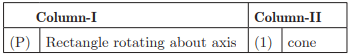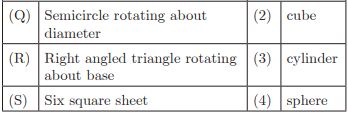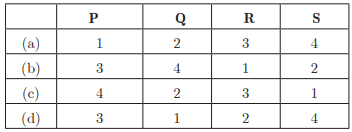Detailed Solution for Test: Surface Area & Volumes- Assertion & Reason Type Questions - Question 10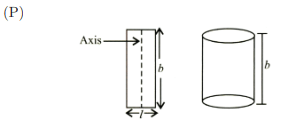When we rotate rectangle about its axis, a cylinder is formed.

(Q) When a semicircle is rotated about its diameter, a sphere is formed.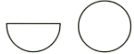(R) When a right angled triangle is rotated about its base, a cone is formed.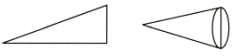(S) Six square sheets are joined to form a cube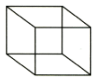## Mathematics for EmSAT Achieve

151 videos|237 docs|254 tests
Information about Test: Surface Area & Volumes- Assertion & Reason Type Questions Page
In this test you can find the Exam questions for Test: Surface Area & Volumes- Assertion & Reason Type Questions solved & explained in the simplest way possible. Besides giving Questions and answers for Test: Surface Area & Volumes- Assertion & Reason Type Questions, EduRev gives you an ample number of Online tests for practice

## Mathematics for EmSAT Achieve

151 videos|237 docs|254 tests